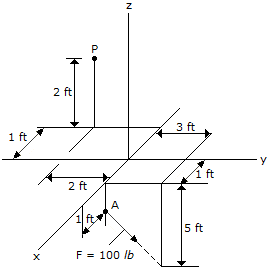Engineering Mechanics - Force System Resultants - Discussion

Discussion :: Force System Resultants - General Questions (Q.No.13)

13.Replace the force at A by an equivalent force and couple moment at P. Express the results on Cartesian vector form.

 [A]. F = (60j-80k) lb, M = (20i+160j+120k) lb-ft [B]. F = (60j-80k) lb, M = (300j+560k) lb-ft [C]. F = (60j-80k) lb, M = 20i lb-ft [D]. F = (60j-80k) lb, M = (-160i-400j+120k) lb-ft

Explanation:

No answer description available for this question.